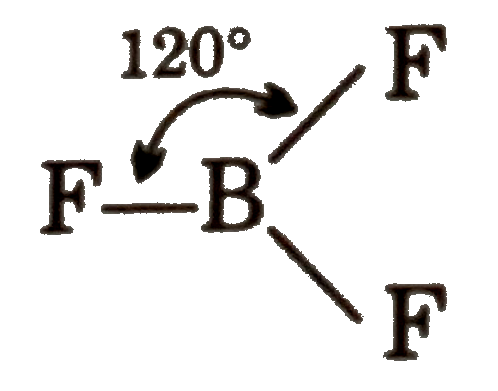# Quantum number is address of an electron in amy atom . They are of four types : (A) Principal quantum number (n) (b) Azimuthal quantum number (l) (c )

33 views

closed
Quantum number is address of an electron in amy atom . They are of four types :
(A) Principal quantum number (n)
(b) Azimuthal quantum number (l)
(c ) Magnetic quantum number (m)
(d) spin quantum number (s)
prinicial quantum tells us the number of shells . Azimuthal quantum number tells is the name o f sub - shell ./ FOr s,p,f:l = 0,1,2,3 respectively .Magnetic quantum number represents the orientation of sub shell and spn wuantum number repesents the quantum spin states .
Elemetn A has principal quantum number 2 for last electron and it has 3 electrons in valence shell and element B has prinvipal quantum number 3 for last electron and it has 7 electrons in avalence shell . the single central atom ) will be :
A. 120^(@)
B. 90^(@)
C. 180^(@)
D. 60^(@)

by (61.2k points)
selected by